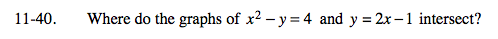Home > A2C > Chapter 11 > Lesson 11.2.1 > Problem11-40

11-40.

Where do the graphs of x2 − y = 4 and y = 2x − 1 intersect? Homework Help ✎Substitute the second equation for y into the first.

(−1, −3) and (3, 5)